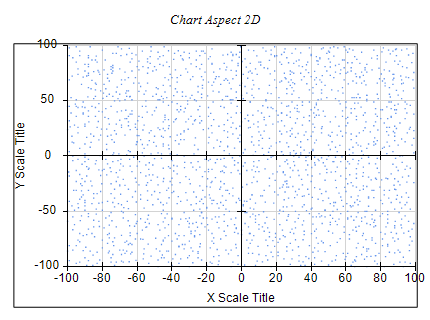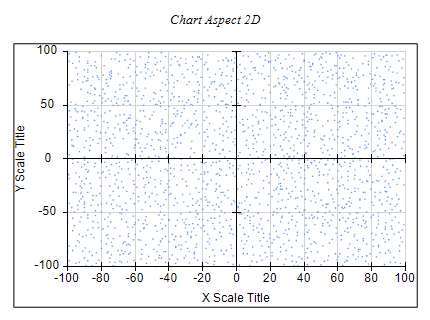﻿ Chart Aspect
Nevron .NET Vision Documentation
Chart Aspect

Chart Aspect in 2D

The Cartesian chart panel aspect in 2D (ratio between plot Width and Height) depends on the following properties:

BoundsMode - controls how the chart fills the available panel content area.

UsePlotAspect - controls whether the chart should use Width / Height properties of the chart panel to calculate the aspect of the chart plot instead of the chart panel.

The following example shows how to create a chart that has a plot aspect of 1:1 (square):

C#
Copy Code
```NCartesianChart chart = (NCartesianChart)nChartControl1.Charts;
chart.BoundsMode = BoundsMode.Fit;
chart.UsePlotAspect = true;
chart.Width = 1;
chart.Height = 1;
```
Visual Basic
Copy Code
```Dim chart As NCartesianChart = CType(NChartControl1.Charts(0), NCartesianChart)
chart.BoundsMode = BoundsMode.Fit
chart.UsePlotAspect = True
chart.Width = 1
chart.Height = 1
```

Fit2DAxisContentMode - controls how the axis labels and titles are fitted inside the panel content bounds. The following table shows the available options:

Fit2DAxisContentMode Description
Disabled Axis content fitting is disabled
Auto Axis content fitting is enabled and both the labels height and width are regarded. Width is regarded only when the axis is not zoomed. This is the default.
LabelsHeight Axis content fitting is enabled however only the labels height is regarded.
LabelsWidthAndHeight Axis content fitting is enabled and both the labels height and width are regarded.

The following code shows how to set the Fit2DAxisContentMode property:

C#
Copy Code
```NCartesianChart chart = (NCartesianChart)nChartControl1.Charts;
chart.Fit2DAxisContentMode = Fit2DAxisContentMode.LabelsHeight;
```
Visual Basic
Copy Code
```Dim chart As NCartesianChart = CType(NChartControl1.Charts(0), NCartesianChart)
chart.Fit2DAxisContentMode = Fit2DAxisContentMode.LabelsHeight
```

The following images show how this property affects the axis fitting in the chart content panel:

Chart with axis fittingChart Aspect in 3D

The chart panel aspect (ratio between width / height and depth) in the case of 3D is controlled from the NChart Width, Height and Depth properties:

C#
Copy Code
```NCartesianChart chart = (NCartesianChart)nChartControl1.Charts;
chart.Enable3D = true;
chart.Width = 50.0f;
chart.Height = 50.0f;
chart.Depth = 50.0f;
```
Visual Basic
Copy Code
```Dim chart As NCartesianChart = CType(NChartControl1.Charts(0), NCartesianChart)
chart.Enable3D = True
chart.Width = 50.0F
chart.Height = 50.0F
chart.Depth = 50.0F
```

Similarly to the case of 2D you can also control whether axis content is fitted inside the chart panel bounds. This is achieved with the Fit3DAxisContent property.

C#
Copy Code
```NCartesianChart chart = (NCartesianChart)nChartControl1.Charts;
chart.Fit3DAxisContent = true;
```
Visual Basic
Copy Code
```Dim chart As NCartesianChart = CType(NChartControl1.Charts(0), NCartesianChart)
chart.Fit3DAxisContent = True
```